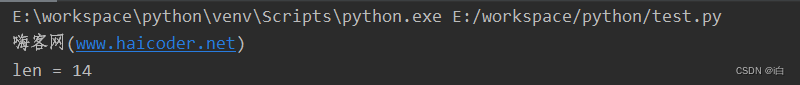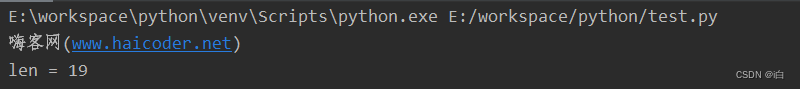# 程序员最近都爱上了这个网站  程序员们快来瞅瞅吧！  it98k网:it98k.com

出租广告位,需要合作请联系站长

+关注

# Python获取字符串长度

## Python获取字符串长度教程

Python 中要想获取 字符串 长度可以使用 len() 函数。

## Python len()函数详解

### 语法

``````len(string)
``````

string要获取长度的字符串。

## 案例

### 获取字符串长度

``````print("嗨客网(www.haicoder.net)")

# 使用 len() 函数，获取字符串的长度
str1 = "Hello,HaiCoder"
print('len =', len(str1))
``````### 获取中文字符串长度

``````print("嗨客网(www.haicoder.net)")

# 使用 len() 函数，获取中文字符串的长度
str1 = "Hello,嗨客网(HaiCoder)"
print('len =', len(str1))
``````## Python获取字符串长度总结

``````len(string)
``````

11 0

pdf(new) 更多>